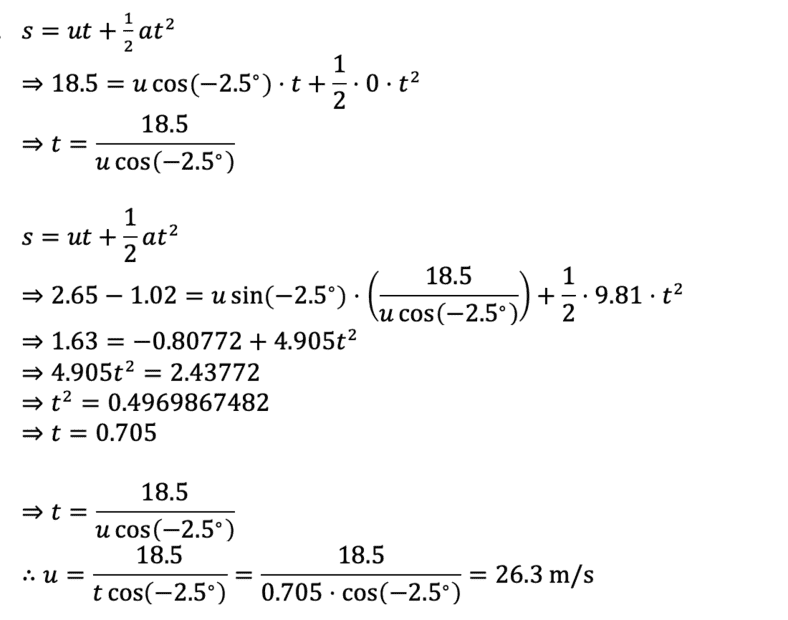# Projectile motion problem – determining initial velocity of throw

Kaiser98
Homework Statement:
A pitcher throws an overhand fastball from an approximate height of 2.65 m and at an
angle of 2.5° below horizontal. The catcher, catches the ball high in the strike zone, at a
height of 1.02 m above the ground. If pitcher's mound and home plate are 18.5 m apart,
what is the initial velocity of the pitch?
Relevant Equations:
s = ut + 0.5 at^2

Answer given in book: 45.2 m/sMy reasoning was to use this kinematic equation to first get time of flight of the baseball using horizontal components, and then use this same equation again to find initial velocity.

Last edited:

Staff Emeritus
Homework Helper
Gold Member
Homework Statement:: A pitcher throws an overhand fastball from an approximate height of 2.65 m and at an
angle of 2.5° below horizontal. The catcher, catches the ball high in the strike zone, at a
height of 1.02 m above the ground. If pitcher's mound and home plate are 18.5 m apart,
what is the initial velocity of the pitch?
Relevant Equations:: s = ut + 0.5 at^2

Answer given in book: 45.2 m/s

View attachment 317812

My reasoning was to use this kinematic equation to first get time of flight of the baseball using horizontal components, and then use this same equation again to find initial velocity.
Hello @Kaiser98 .You are inconsistent with your use of signs.

You have vertical distance the ball drops as being positive as well as acceleration due to gravity as being positive. That's all fine.
However, you have the angle below horizontal as being negative. Since you had been treating the downward direction as being positive for the other quantities, so should the 2.5° angle be positive.

•PeroK and Kaiser98
••